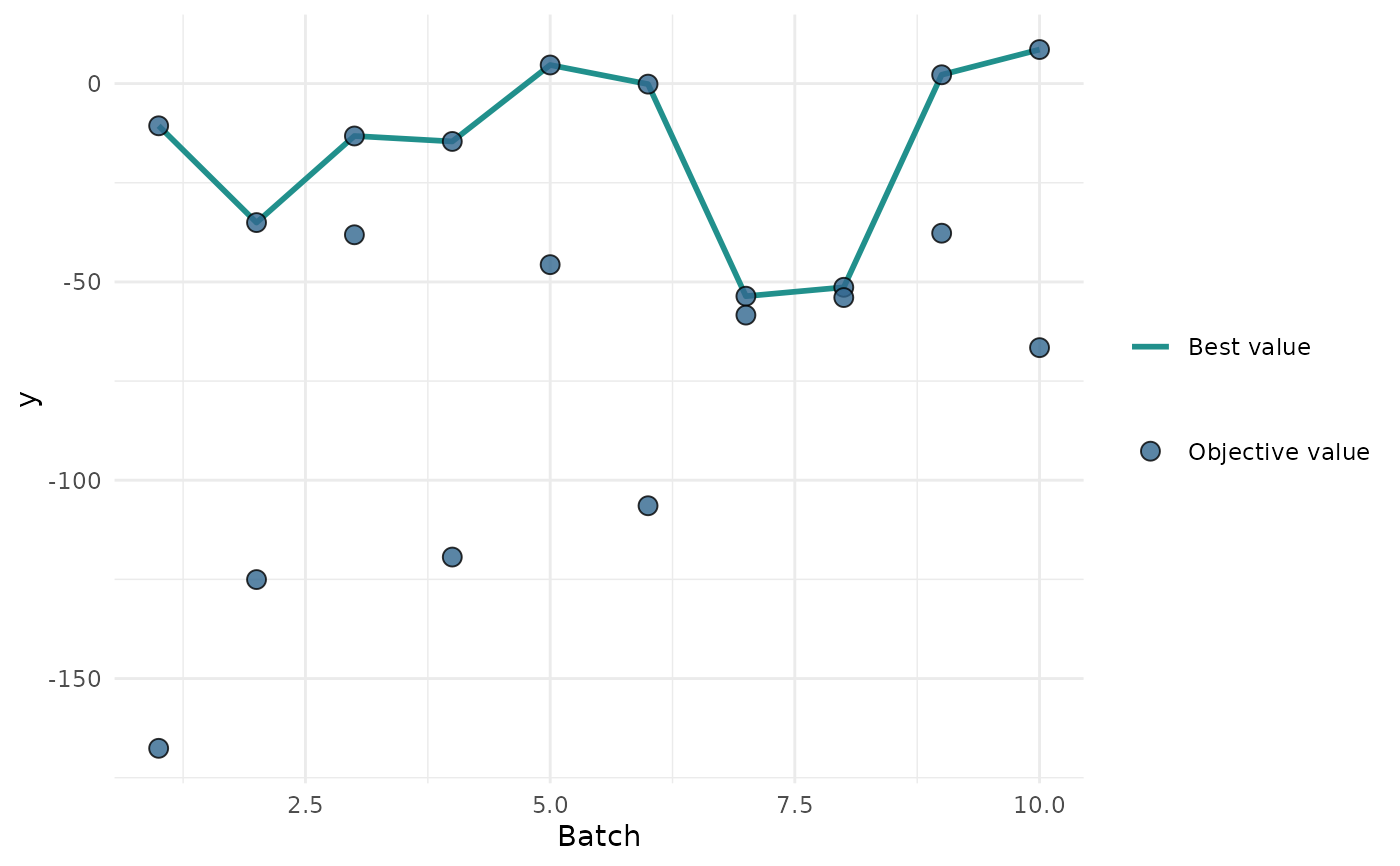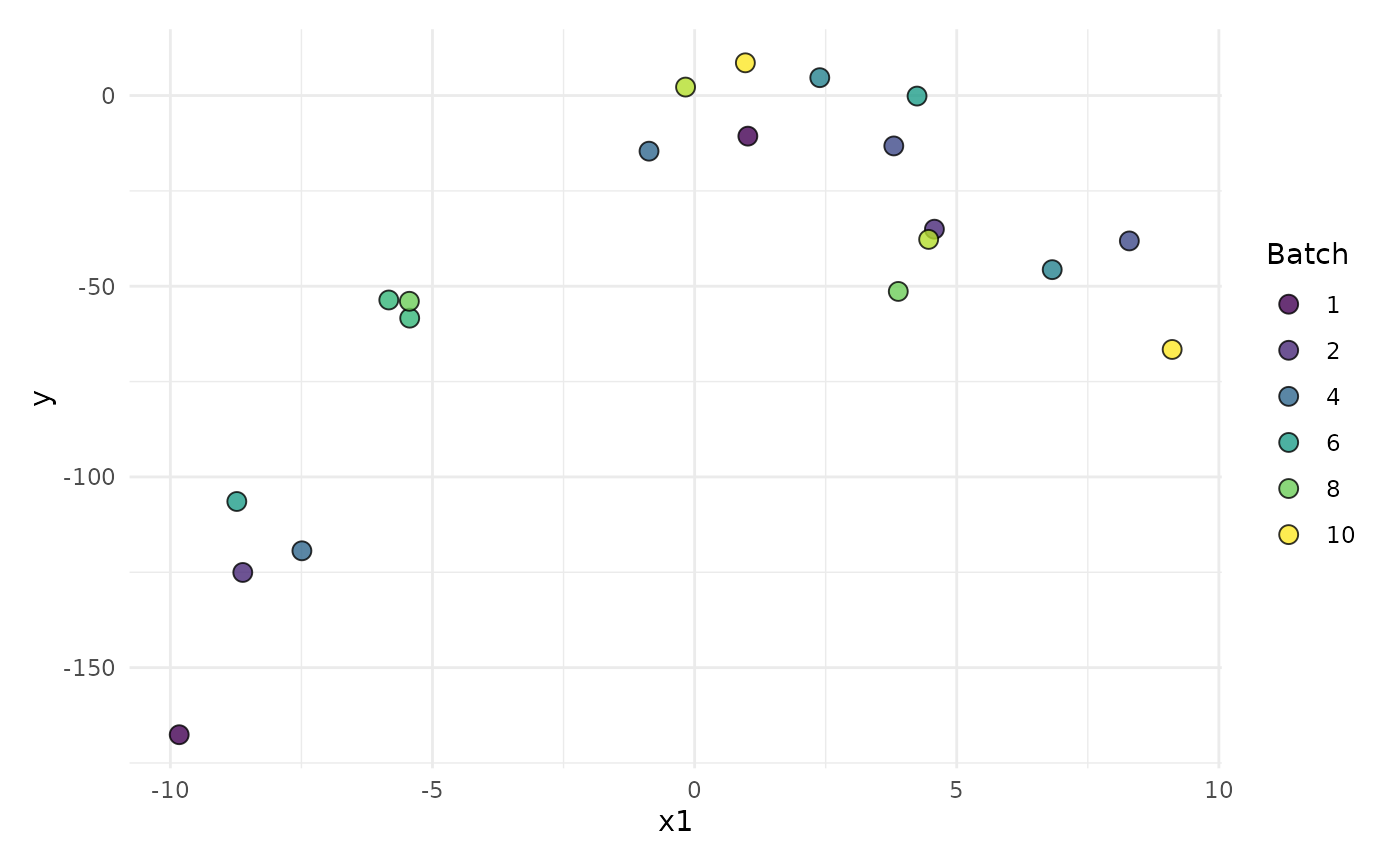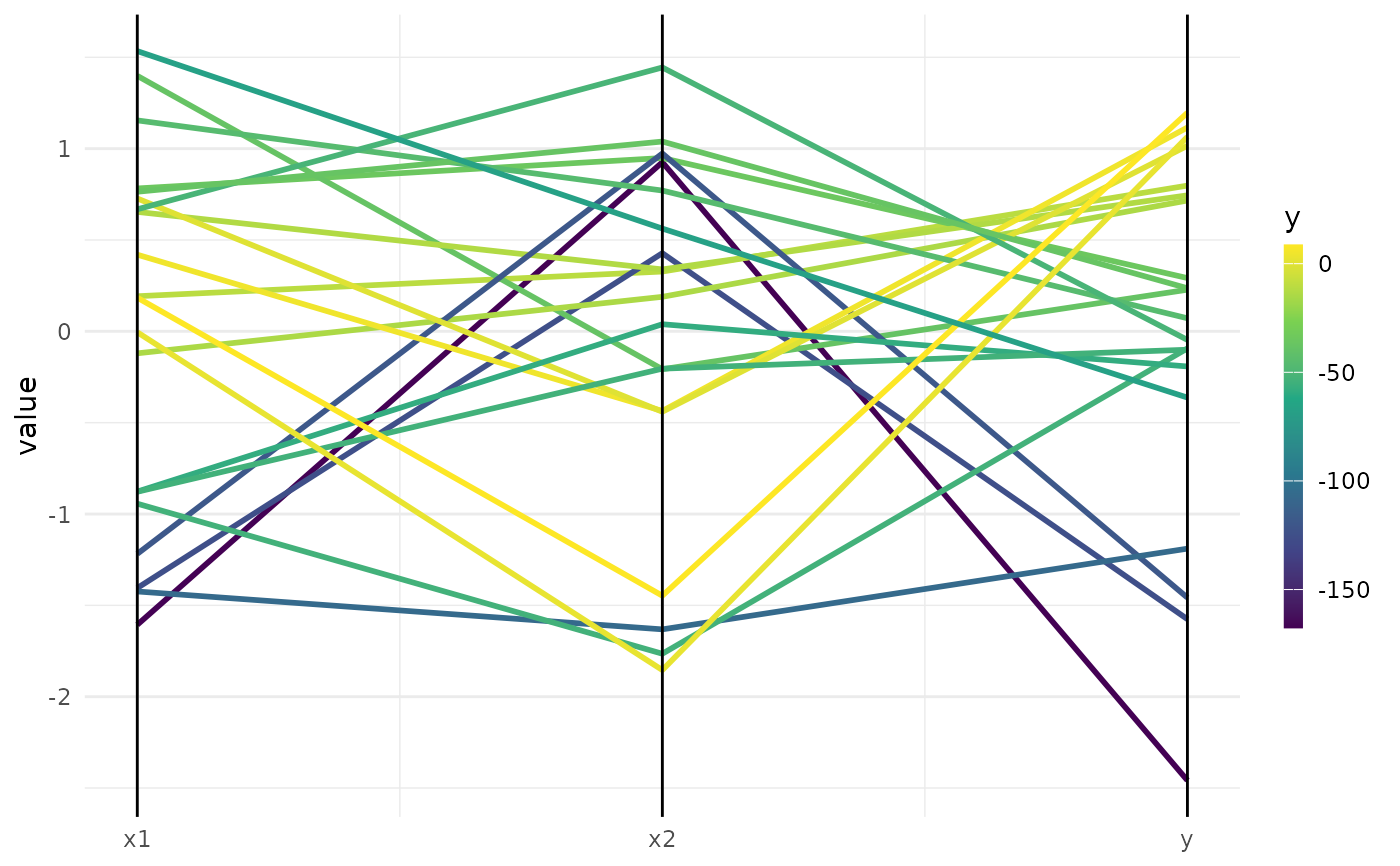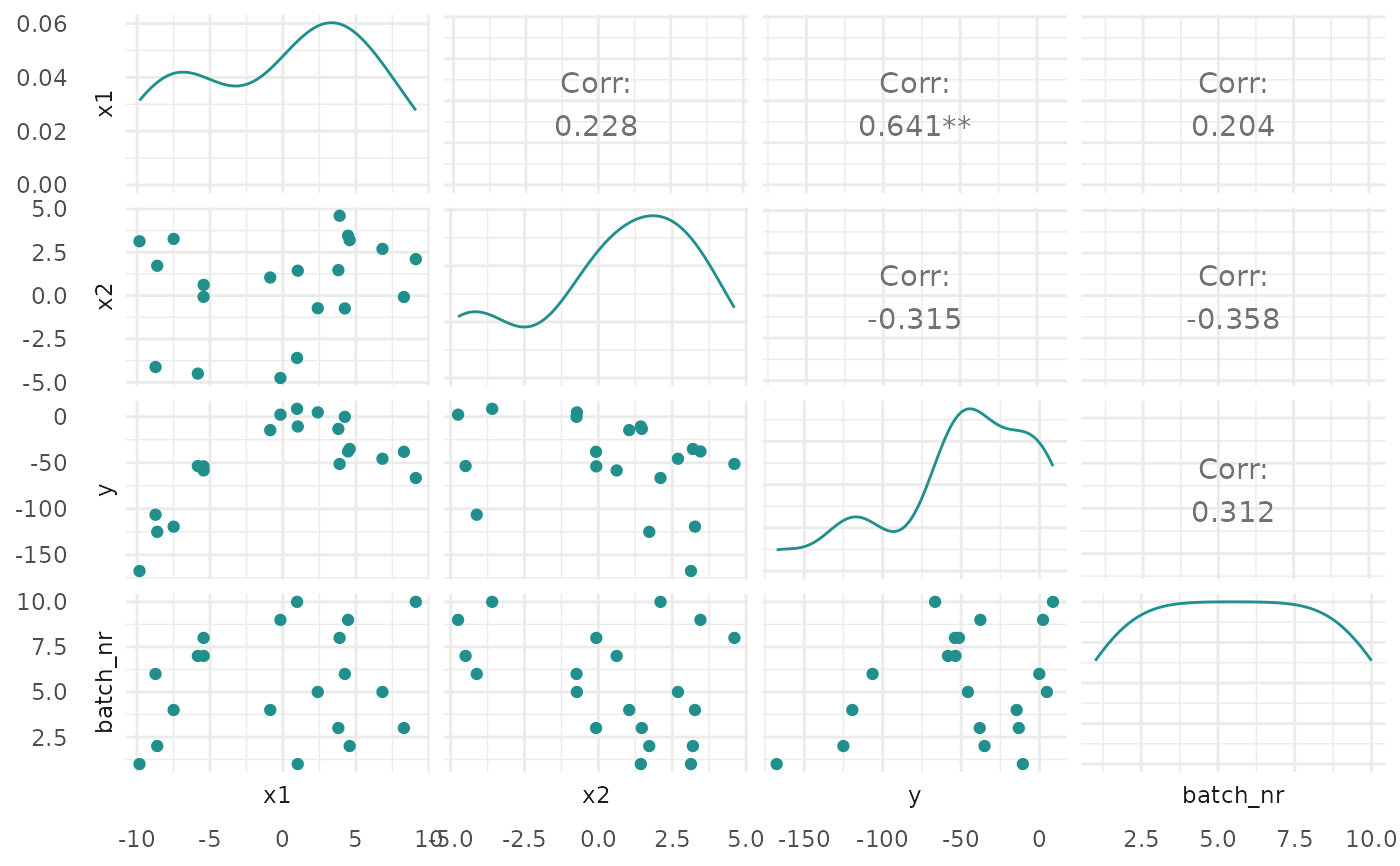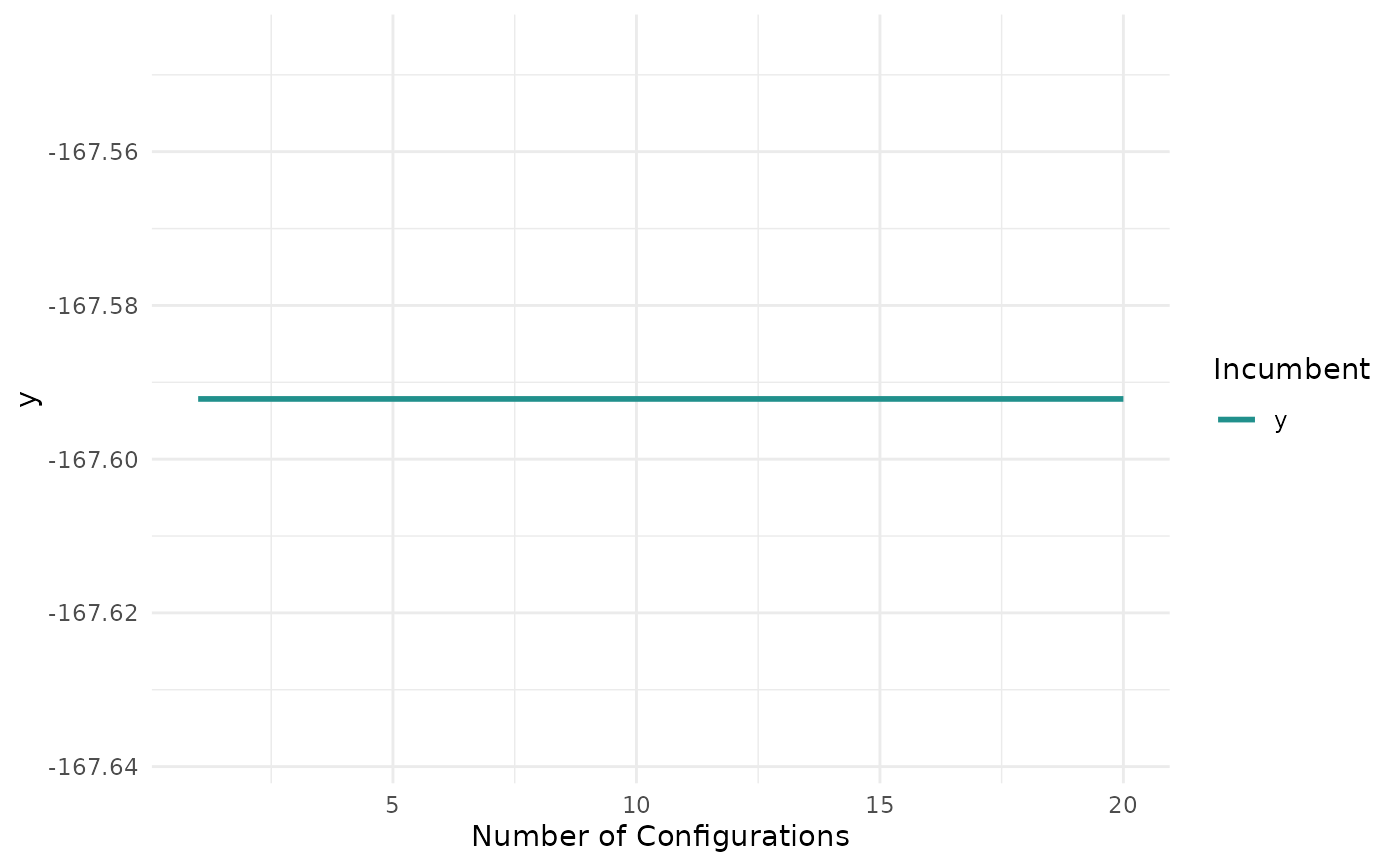Visualizations for bbotk::OptimInstanceSingleCrit. The argument type controls what kind of plot is drawn. Possible choices are:

• "marginal" (default): Scatter plots of x versus y. The color of the points shows the batch number.

• "performance": Scatter plots of batch number versus y

• "parameter": Scatter plots of batch number versus input. The color of the points shows the y values.

• "parallel": Parallel coordinates plot. x values are rescaled by (x - mean(x)) / sd(x).

• "points": Scatter plot of two x dimensions versus. The color of the points shows the y values.

• "surface": Surface plot of two x dimensions versus y values. The y values are interpolated with the supplied mlr3::Learner.

• "pairs": Plots all x and y values against each other.

• "incumbent": Plots the incumbent versus the number of configurations.

## Usage

# S3 method for OptimInstanceSingleCrit
autoplot(
object,
type = "marginal",
cols_x = NULL,
trafo = FALSE,
learner = mlr3::lrn("regr.ranger"),
grid_resolution = 100,
batch = NULL,
theme = theme_minimal(),
...
)

## Arguments

object
type

(character(1)):
Type of the plot. See description.

cols_x

(character())
Column names of x values. By default, all untransformed x values from the search space are plotted. Transformed hyperparameters are prefixed with x_domain_.

trafo

(logical(1))
If FALSE (default), the untransformed x values are plotted. If TRUE, the transformed x values are plotted.

learner

(mlr3::Learner)
Regression learner used to interpolate the data of the surface plot.

grid_resolution

(numeric())
Resolution of the surface plot.

batch

(integer())
The batch number(s) to limit the plot to. The default is all batches.

theme

(ggplot2::theme())
The ggplot2::theme_minimal() is applied by default to all plots.

...

(ignored).

## Value

ggplot2::ggplot().

## Examples

if (requireNamespace("mlr3") && requireNamespace("bbotk") && requireNamespace("patchwork")) {
library(bbotk)

fun = function(xs) {
c(y = -(xs[] - 2)^2 - (xs[] + 3)^2 + 10)
}
domain = ps(
x1 = p_dbl(-10, 10),
x2 = p_dbl(-5, 5)
)
codomain = ps(
y = p_dbl(tags = "maximize")
)
obfun = ObjectiveRFun$new( fun = fun, domain = domain, codomain = codomain ) instance = OptimInstanceSingleCrit$new(objective = obfun, terminator = trm("evals", n_evals = 20))

optimizer = opt("random_search", batch_size = 2)
optimizer\$optimize(instance)

# plot y versus batch number
print(autoplot(instance, type = "performance"))

# plot x1 values versus performance
print(autoplot(instance, type = "marginal", cols_x = "x1"))

# plot parallel coordinates plot
print(autoplot(instance, type = "parallel"))

# plot pairs
print(autoplot(instance, type = "pairs"))

# plot incumbent
print(autoplot(instance, type = "incumbent"))
}